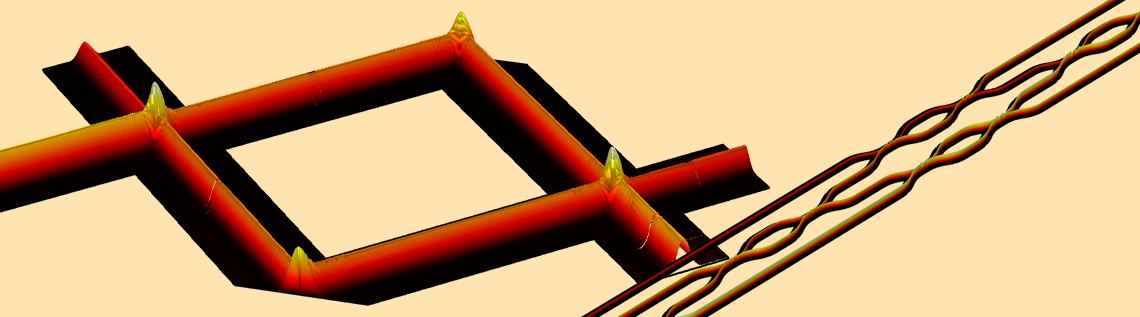# 通过仿真分析光学计算设备

2022年 8月 11日

### 光学矩阵乘法

#### 马赫-曾德尔调制器

E= \begin
{bmatrix}E_1 \\E_2\end{bmatrix},
E’= \begin
{bmatrix}E’_1 \\E’_2\end{bmatrix}

U^2(\theta, \alpha, \beta) 是任意酉矩阵，由 \theta, \alpha\beta. 控制。 这里，上标 2 表示矩阵的维数。我们将在整篇文章中遵循这个符号约定。通过控制 \theta, \alpha\beta 我们可以让这个光学系统以光速进行任何单一的 2×2 矩阵乘法。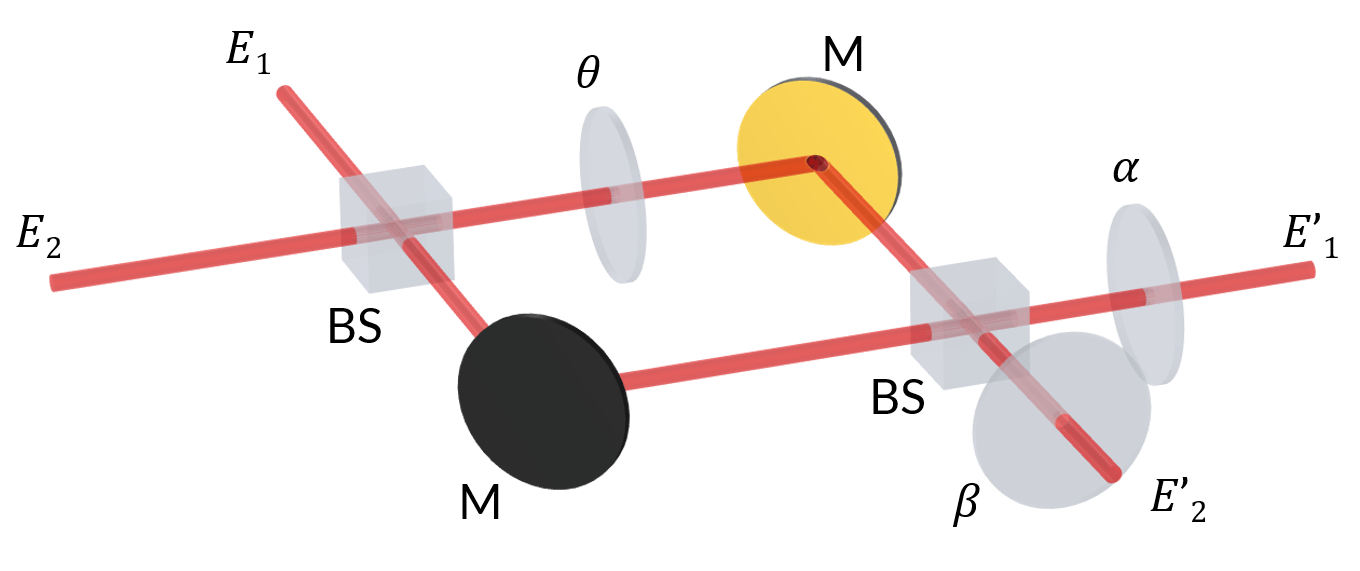E_1
。反射光束中虚数 -j 的出现是由于反射相移 \pi/2，因为 e^{-j\pi/2}=-j。 对于通过分束器的光，比如说第一个分束器，它会拾取一个相位因子 e^{-j\theta}。 根据以上讨论的信息，我们可以对经过不同路径的光求 E’_1E’_2，得出和：

E’_1=j\frac{\sqrt{2}}

{2}(-j\frac{\sqrt{2}}{2}
E_1+\frac{\sqrt{2}}

{2}E_2)e^{j\theta}e^{-j\alpha}\frac{\sqrt{2}}{2}
(\frac{\sqrt{2}}

{2}E_1-j\frac{\sqrt{2}}{2}
E_2)e^{-j\alpha},

E’_2=-\frac{\sqrt{2}}

{2}(-j\frac{\sqrt{2}}{2}
E_1+\frac{\sqrt{2}}

{2}E_2)e^{-j\theta}e^{-j\beta}+j\frac{\sqrt{2}}{2}
(\frac{\sqrt{2}}

{2}E_1-j\frac{\sqrt{2}}{2}
E_2)e^{-j\beta}

E’_1=\frac{e^{-j\alpha}}

{2}[(e^{-j\theta}-1)E_1+j(1+e^{-j\theta})E_2],

E’_2=\frac{e^{-j\beta}}{2}
[j(1+e^{-j\theta})E_1+(1-e^{-j\theta})E_2]

\begin

{bmatrix}E’_1\\E’_2\end{bmatrix}
=\frac

{1} {2} \begin{bmatrix}e^{-j\alpha}(e^{-j\theta}-1) & je^{-j\alpha}(1+e^{-j\theta})\\je^{-j\beta}(1+e^{-j\theta}) & e^{-j\beta}(1-e^{-j\theta})\end{bmatrix} \begin{bmatrix}E_1\\E_2\end{bmatrix}

U^2(\theta,\alpha,\beta)=\begin{bmatrix}e^{-j\alpha}(e^{-j\theta}-1) & je^{-j\alpha}(1+e^{-j\theta})\\je^{-j\beta}(1+e^{-j\theta}) & e^{-j\beta}(1-e^{-j\theta})\end{bmatrix}

• 第一个波：
• x = ewbe.k
• y = 0
• 第二个波：
• x = 0
• y = -ewbe.k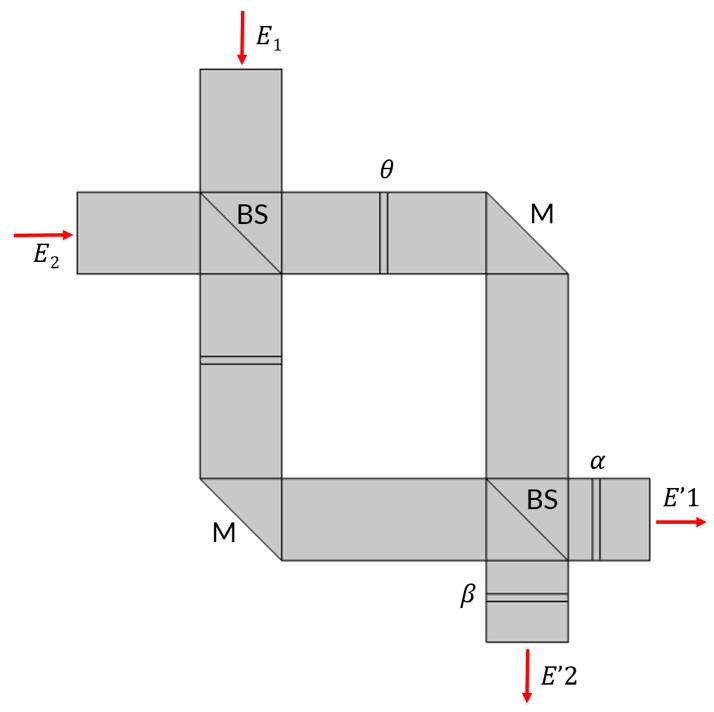​​​左图：经典马赫曾德尔调制器的二维模型，具有两个 50:50 分束器 (BS) 和三个移相器，可将光的相位移动 \theta, \alpha\beta。M 表示反射镜。右图：模拟 \theta 从 0 到 2\pi变化的场分布。 输入振幅度 E_1=1V/mE_2=2V/m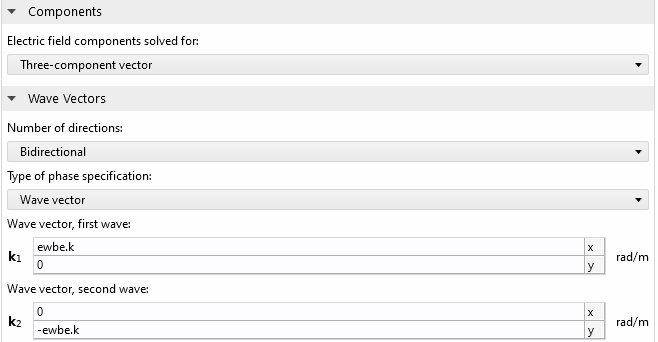\theta 从 0 到变化 2\pi 时，复数 E’_1(左图)和 E’_2(右图)。实线表示模拟结果，星号表示使用上述解析推导计算的期望值。横轴和纵轴分别是实部和虚部，颜色代表 \theta 的变化。

#### n×n 酉矩阵乘法

R_{11} = \begin{bmatrix} 1 & 0 & 0\\ 0 & 1 & 0\\ 0 & 0 & U^2_1 \end{bmatrix}
R_{22} = \begin{bmatrix} 1 & 0 & 0\\ 0 & U^2_2 & 0\\ 0 & 0 & 1 \end{bmatrix}
R_{21} = \begin{bmatrix} 1 & 0 & 0\\ 0 & 1 & 0\\ 0 & 0 & U^2_3 \end{bmatrix}
R_{33} = \begin{bmatrix} U^2_4 & 0 & 0\\ 0 & 1 & 0\\ 0 & 0 & 1 \end{bmatrix}
R_{32} = \begin{bmatrix} 1 & 0 & 0\\ 0 & U^2_5 & 0\\ 0 & 0 & 1 \end{bmatrix}
R_{31} = \begin{bmatrix} 1 & 0 & 0\\ 0 & 1 & 0\\ 0 & 0 & U^2_6 \end{bmatrix}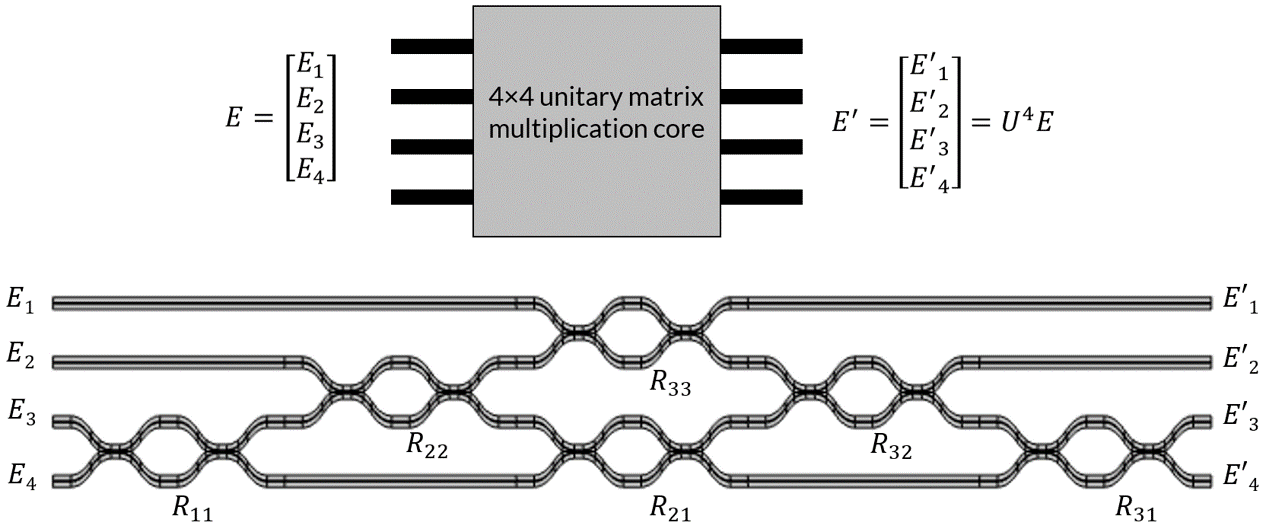#### 广义 n×m 矩阵乘法### 拓展资源

• 想了解更多关于摩尔定律、光计算和马赫-曾德尔调制器网络的信息吗？请查看以下这些相关资源。

### 参考文献

1. J. Cheng, H. Zhou, and J. Dong, “Photonic Matrix Computing: From Fundamentals to Applications”, Nanomaterials, 11(7), 1683, 2021.